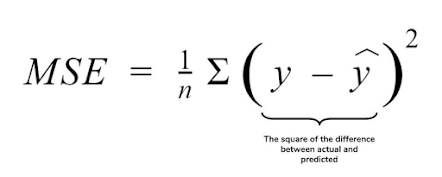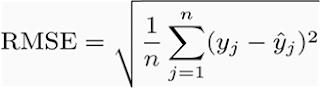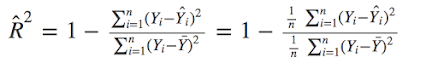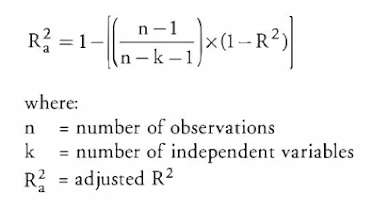# Know the best Evaluation Metrics for the Regression Models

Hello, In this article we are going to look over each and every metric used for evaluating machine learning regression models, and put some light on each metric. And after all, we will try to figure out which metrics will best according to the problem statement you are dealing with.

The idea of building Machine learning models works on the constructive feedback principle. You build a model, get feedback from metrics, improve performance and continue until you achieve a desirable accuracy. Evaluation metrics help in understanding the performance of the model.

Before starting let's see what is Error in this context, simply the error is calculated as the difference between actual and predicted values. If you want to learn more about the Regression terms then please read the complete article on Regression Analysis to overcome all your doubts.

Plenty of analysis and aspiring data scientists most of the time do not bother about how robust is their model is. Once they are finished with modeling, they hurriedly map predicted values on unseen data. This is an incorrect approach.

🔖 Simply building a predictive model is not your motive. It's about creating and selecting the right model that exhibits high accuracy and does not overfit the data. Hence, it is crucial to check the accuracy of your model before computing the predicted values.

🔖 In most cases, we only used or come across MSE, R2, or MAE metrics for Regression purposes. but there are many more metrics that are important not only in development but also as interview points of view. When you explore different data science opportunities in the corporate world through open-source competitions, machine learning hackathons, Datathon, etc then they will ask you to use different evaluation metrics in different use-case so Is important to be aware of important and mostly used evaluation metrics.

## Different Evaluation Metrics for Regression

Now let's learn about different Evaluation metrics we have seen so far in the table of contents above. And we will also pick their advantages and disadvantages to understand which to use according to a problem statement.

### 1) MSE (Mean Squared Error)

MSE or mean squared error is one of the most used metrics for each regression task. It is very simple to understand that It is simply the average of the squared difference between the target value and predicted value by model.

In simple words, we find the error(Actual - Predicted) of each observation, and to demonstrate the total error of the complete dataset we find the mean(average) of all the errors to avoid the cancellation of positive and negative terms in error we basically square them all and get the final result as mean squared error.

As it is a squared difference, it penalizes small errors which lead to over-estimation of how bad the model performs.1. It is easily differentiable and hence, can be optimized better. And this is the biggest benefit of using MSE.
1. The unit is not the same as the output. you have to find the square root of the predicted value for seeing the actual value in a loss.
2. It is not robust to outliers.

### 2) MAE(Mean Absolute Error)

MAE is the average of the absolute difference between the actual and predicted value and it is divided by the total number of differences. The MAE plays a more robust role towards the outliers and does not penalize the error as done by MSE.

MAE is a linear score that means all individual differences are weighted equally in average. for example, the difference between 10 and 0 will be twice the difference between 5 and 0. The same is not true for RMSE which we will discuss in further detail.

1. The output that this metric gives is in the same unit as input like input is in lakh so the output will be also in lakhs.
2. It is Robust to outliers.

1. The Graph is not differentiable at Zero and that's why we have MSE which we have already seen.

### 3) RMSE(Root Mean Squared Error)

One problem with MSE is that, we are taking the square of errors, it changes the units. for example, I am having an observation in meter(m) like 19 and 28 and if I calculate the MSE of complete data then it will be much high because now, the unit will be meter squared(m^2).
So, to overcome this problem we can take the square root of the calculated MSE to get back the actual observation and unit.Listen, Might RMSE would also not be a good metric for model evaluation. Wondering why? Let's see this by understanding the next metric as RMSLE.

1. The output is in the same unit.
2. The metric is mostly used in the deep learning problem statement.
1. It is also not robust to outliers.

### 4) RMSLE(Root Mean Squared Log Error)

suppose I have 2 observations:
Case-1:   actual = 1             and       predicted=401
Case-2:   actual = 10,001    and       predicted = 10,401

Now, something has really gone wrong with the model and it gives a decent performance. And If I calculate RMSE in both cases it will be 282. So, in such a problem, if we can't use RMSE then, what we should do is instead of directly taking the average of the squared error we can take the log of the values and then take the average of the square of their values.

Using log basically scales down the larger values. so, RMSLE in the first case is 2.65 and in case 2 it is 0.0014. So, now we know that model is performing decently well.

### 5) R Squared (R2)

We discuss 4 metrics for regression and also saw that if MSE or RMSE decreases then model performance increases. But these metrics alone are not intuitive. why? let's have a look at that.

In the classification problem, we are having a benchmark to compare our model against because the accuracy of which is 0.5 and the random model here can be treated here as a benchmark. But when we talk about MSE or RMSE then, we do not have any benchmark to compare our model against.
So, can there be any benchmark in the regression problem where we can compare our model against it? Yes! There is.

Recall the concept of MSE we have discussed above. here we simply take the sum of squared error, instead of looking at it alone we take it with the MSE of the baseline model. means we should divide the MSE of the model with the MSE of the baseline model.

#### Now, what is the baseline model?

It is basically when all the predicted values are simply replaced with the mean of all values. As, you can see that 1/N will cancel out each other, and we will be left out with the sum of squared errors which is called Relative errors.

When the MSE of the model and baseline model is equal, which means that model is predicting the best as predicting the average of values, and if it is more than 1 then, a model is worse than the baseline model. So you might have guessed that the value should be between 0 and 1.

Now, when MSE decreases, the whole value decreases, model performance improves which is just contradicting to the point that higher values are good, so there is a solution for this in form of R2 that subtract the whole value from 1 and known as R Squared. so better is model higher is R squared.#### Problem with R Squared

If we add more feature to the data, then R Squared increases or remains constant, which means it does not decrease regardless of any feature how any feature affect the model. To deal with this issue we have a better version of R Squared known as Adjusted R squared.

### 6) Adjusted R Squared

It takes the number of samples into account and adds more features, the denominator increases, which means the whole fraction increases. Now, if Adjusted R squared increases significantly the, change in R squared can be compensated. And if fraction increases and R squared does not change then, we are subtracting the larger value from 1 and thus, Adjusted R squared could decrease.Now, that we have a metric for regression problems that compare the model with the benchmark and provide a score between 0 and 1.

## Conclusion

Model Evaluation leads a Machine Learning Engineer, Data Scientist, or Practitioner in the right direction to choose or tune a model with the right set of parameters. Every beginner should know these Regression metrics and try to evaluate the model on different metrics and build a robust model to present in your data science folio.
In an interview, knowing this formula and terms will help to discuss the problem statement in an efficient way given to you. If you have any queries then, please post in the comment section below. I will be happy to help you out and be a part of your Data Science journey.

Thank you!..
Keep Learning, Happy learning.